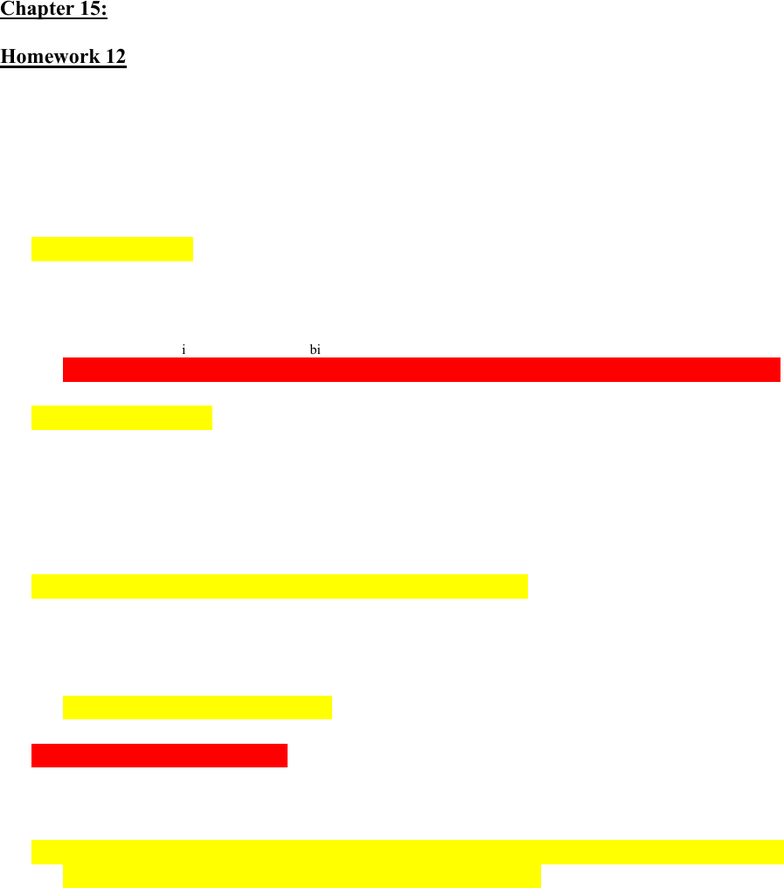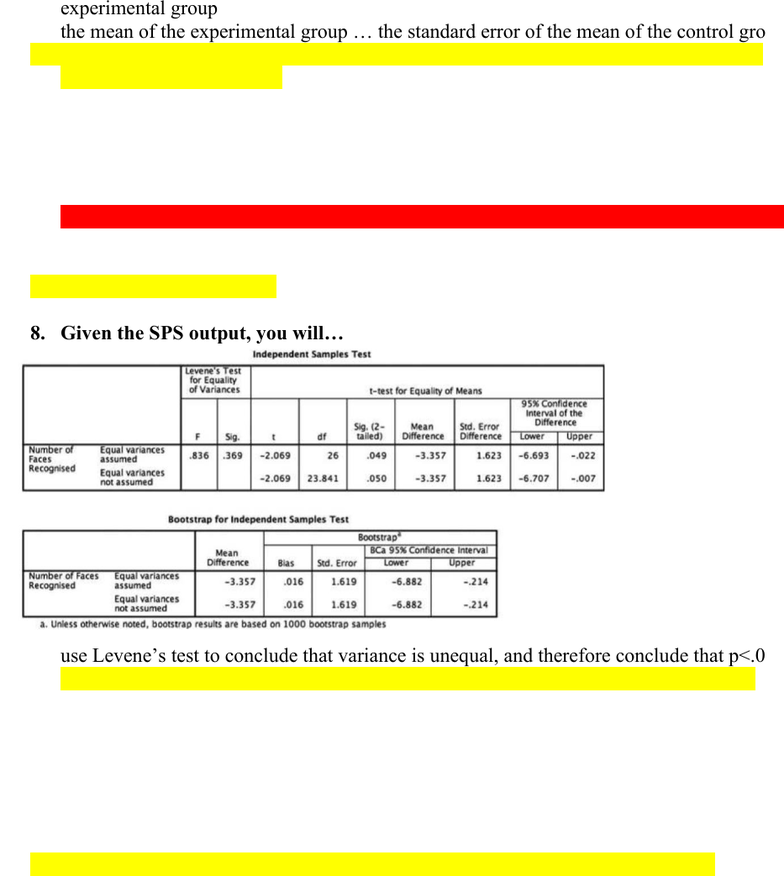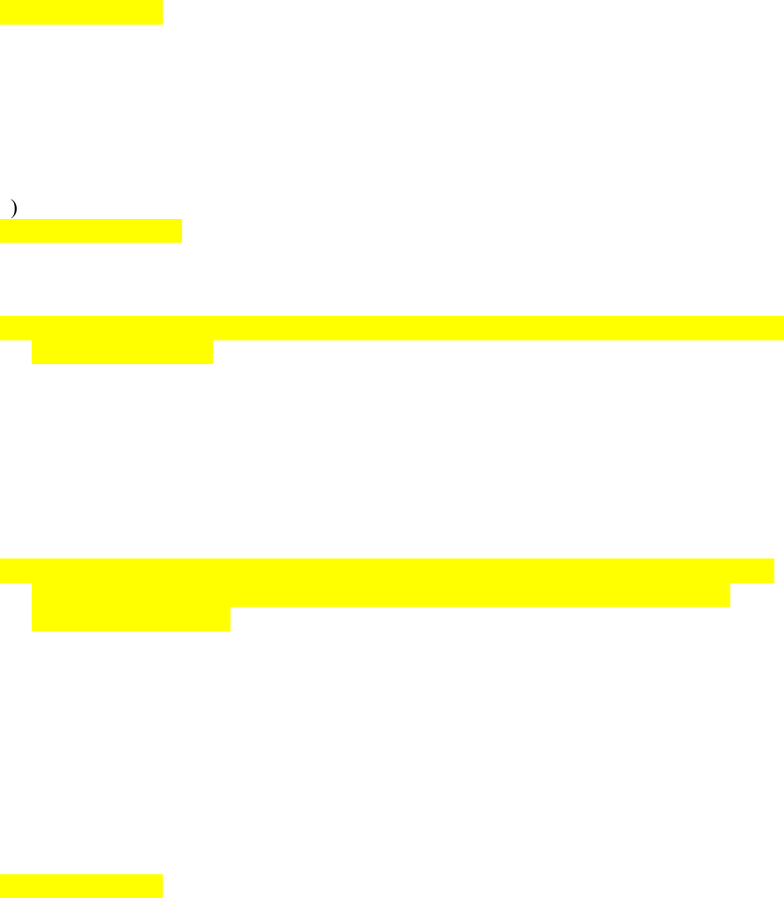# PNB 3XE3 Study Guide - Quiz Guide: Dependent And Independent Variables, Regression Analysis, Confidence Interval

33 views9 pagesChapter 15:
Homework 12
1. In which type of t-test would one participant contribute two data points, which
would then be contrasted with each other?
a) dependent t-test
b) paired samples t-test
c) matched pairs t-test
d) all of the above
2. Which of the following is false regarding the t-test?
a) it is equal to bi divided by SEbi
b) it is equal to mean of X1 mean of X0 divided by the standard error of that difference
c) it can be used to test tests whether the difference between means in 0
d) none of the above
3. A t-test is…
a) a linear model with a dichotomous predictor and a categorical outcome variable
b) not a linear model
c) a linear model with a dichotomous outcome variable
d) a linear model with one dichotomous predictor variable
4. In a t-test, what tells you the expected difference between the means?
a) the standard error of the mean
b) the standard error of differences
c) the observed differences between sample means
d) all of the above are correct
5. Dummy coding is …
a) a way of recoding a categorical variable with two or more categories into one or more
variables that are dichotomous with values of only 0 or 1
b) a short cut that makes t-tests faster
c) use in paired t-tests, but not independent t-tests
d) all of the above
Unlock document

This preview shows pages 1-3 of the document.
Unlock all 9 pages and 3 million more documents.6. In a t-test, the t is a tests statistic representing the signal to noise ratio. The signal is
___ and the noise is ___.
a) the mean of the experimental group … the standard error of the mean of either of the
groups
b) the difference between the means of X1 and X2 … the standard error of the mean of the
experimental group
c) the mean of the experimental group the standard error of the mean of the control group
d) the difference between the means of X1 and X2 … the standard error of the mean of the
differences between groups
7. In a t-test, how would you know whether the difference between your means is big
or small?
a) you evaluate it in the context of the standard error of the mean
b) calculate a t-value and compare it to the critical t-value based on your degrees of freedom
c) compare the difference to an estimate of the standard error of differences in your
measurements
d) all of the above are correct
8. Given the SPS output, you will…
a) use Levene’s test to conclude that variance is unequal, and therefore conclude that p<.05
b) use Levene’s test to conclude that variance is equal, and therefore conclude that p<.05
c) Use Levene’s test to conclude that variance is unequal, and therefore conclude that p =
.05
d) use Levene’s test to conclude that variance is equal, and therefore conclude that p =.05
9. In a t-test, what is the null hypothesis?
a) the means of the two sets of scores are correlated
b) there is no difference in the means of the outcome variable across the two groups
c) knowing which group a case belongs to makes you better able to predict the outcome
score for that case
d) the means of the outcome variable are different across the two groups
Unlock document

This preview shows pages 1-3 of the document.
Unlock all 9 pages and 3 million more documents.10. What is problematic about a median split?
a) two cases that are very similar could be treated as extremely different
b) two cases that are extremely different could be treated as very similar
c) effect sizes get smaller
d) all of the above
11. A t-test in which each of the two samples have no influence on the performance of
the other is called
a) paired-samples t-test
b) dependent t-test
c) matched pairs t-test
d) any of the above
e) independent t-test
12. If your independent sample t-test is significant, this suggests …
a) you are better off predicting the outcome based on group membership than you would be
using the grand mean
b) the two groups are likely different samples of the same population
c) the means of the two sets of scores are correlated
d) you are better off predicting the outcome based on the grand mean than you are using the
group mean
13. How is it possible to calculate the slope b1 for a t-test when the value of the
independent variable is a category membership?
a) the control group is coded with the dummy code 0, and the experimental group is coded
with the dummy code 1. then you can treat 0 and 1 as the levels of the independent
variable in the equation
b) simply insert the category labels into the equation
c) it isn’t, the t-test takes a fundamentally different approach
d) you can convert each category label to a numeric value that makes sense in light of your
prediction
14. The following equation is true: b1 = SCP / SSx
a) for the t-test
b) for the regression analysis
c) for the general linear model
d) all of the above
Unlock document

This preview shows pages 1-3 of the document.
Unlock all 9 pages and 3 million more documents.